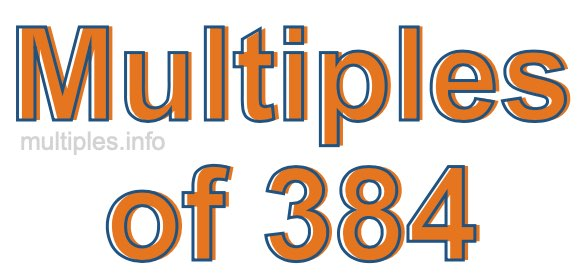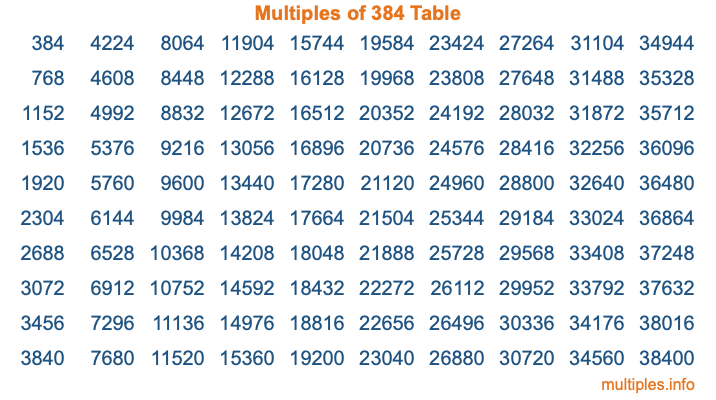Multiples of 384Welcome to the Multiples of 384 page. Here we will first teach you everything you will ever need to know about the multiples of 384, and then give you a study guide summary of everything we taught you to make sure you remember it all. Use this page to look up facts and learn information about the multiples of 384. This page will make you a multiples of three hundred eighty-four expert!

Definition of Multiples of 384
Multiples of 384 are all the numbers that when divided by 384 equal an integer. Each of the multiples of 384 are called a multiple. A multiple of 384 is created by multiplying 384 by an integer.

Therefore, to create a list of multiples of 384, you start with 1 multiplied by 384, then 2 multiplied by 384, then 3 multiplied by 384, and so on for as long as you want. Thus, the list of the first five multiples of 384 is 384, 768, 1152, 1536, and 1920. To see a larger list of multiples of 384, see the printable image of Multiples of 384 further down on this page. We also have a category where you can choose any nth multiple of 384.

Multiples of 384 Checker
The Multiples of 384 Checker below checks to see if any number of your choice is a multiple of 384. In other words, it checks to see if there is any number (integer) that when multiplied by 384 will equal your number. To do that, we divide your number by 384. If the the quotient is an integer, then your number is a multiple of 384.

Is  a multiple of 384?

Least Common Multiple of 384 and ...
A Least Common Multiple (LCM) is the lowest multiple that two or more numbers have in common. This is also called the smallest common multiple or lowest common multiple and is useful to know when you are adding our subtracting fractions. Enter one or more numbers below (384 is already entered) to find the LCM.

Check out our LCM Calculator if you need more details about the Least Common Multiple or if you need the LCM for different numbers for adding and subtraction fractions.

nth Multiple of 384
As we stated above, 384 is the first multiple of 384, 768 is the second multiple of 384, 1152 is the third multiple of 384, and so on. Enter a number below to find the nth multiple of 384.

th multiple of 384

Multiples of 384 vs Factors of 384
384 is a multiple of 384 and a factor of 384, but that is where the similarities end. All postive multiples of 384 are 384 or greater than 384. All positive factors of 384 are 384 or less than 384.

Below is the beginning list of multiples of 384 and the factors of 384 so you can compare:

Multiples of 384: 384, 768, 1152, 1536, 1920, etc.

Factors of 384: 1, 2, 3, 4, 6, 8, 12, 16, 24, 32, 48, 64, 96, 128, 192, 384

As you can see, the multiples of 384 are all the numbers that you can divide by 384 to get a whole number. The factors of 384, on the other hand, are all the whole numbers that you can multiply by another whole number to get 384.

It's also interesting to note that if a number (x) is a factor of 384, then 384 will also be a multiple of that number (x).

Multiples of 384 vs Divisors of 384
The divisors of 384 are all the integers that 384 can be divided by evenly. Below is a list of the divisors of 384.

Divisors of 384: 1, 2, 3, 4, 6, 8, 12, 16, 24, 32, 48, 64, 96, 128, 192, 384

The interesting thing to note here is that if you take any multiple of 384 and divide it by a divisor of 384, you will see that the quotient is an integer.

Multiples of 384 Table
Below is an image of the first 100 multiples of 384 in a table. The table is in chronological order, column by column. The first column has the first ten multiples of 384, the second column has the next ten multiples of 384, and so on.The Multiples of 384 Table is also referred to as the 384 Times Table or Times Table of 384. You are welcome to print out our table for your studies.

Negative Multiples of 384
Although not often discussed or needed in math, it is worth mentioning that you can make a list of negative multiples of 384 by multiplying 384 by -1, then by -2, then by -3, and so on, to get the following list of negative multiples of 384:

-384, -768, -1152, -1536, -1920, etc.

Multiples of 384 Summary
Below is a summary of important Multiples of 384 facts that we have discussed on this page. To retain the knowledge on this page, we recommend that you read through the summary and explain to yourself or a study partner why they hold true.

There are an infinite number of multiples of 384.

A multiple of 384 divided by 384 will equal a whole number.

384 divided by a factor of 384 equals a divisor of 384.

The nth multiple of 384 is n times 384.

The largest factor of 384 is equal to the first positive multiple of 384.

384 is a multiple of every factor of 384.

384 is a multiple of 384.

A multiple of 384 divided by a divisor of 384 equals an integer.

384 divided by a divisor of 384 equals a factor of 384.

Any integer times 384 will equal a multiple of 384.

Multiples of a Number
Here you can get the multiples of another number, all with the same attention to detail as we did for multiples of 384 on this page.

Multiples of
Multiples of 385
Did you find our page about multiples of three hundred eighty-four educational? Do you want more knowledge? Check out the multiples of the next number on our list!